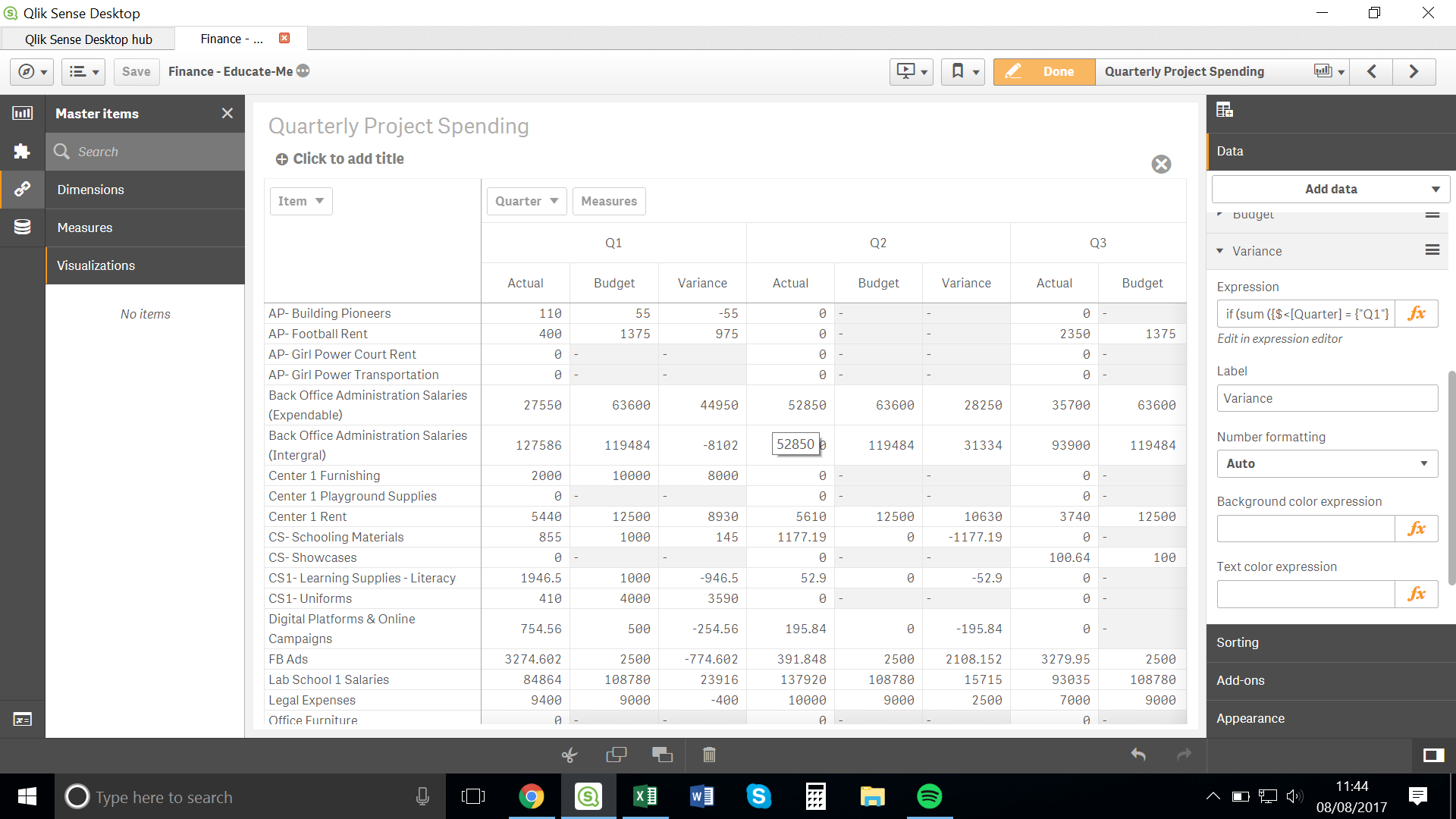New to Qlik Sense

Discussion board where members can get started with Qlik Sense.

AnnouncementsNot applicable

Quarterly Variance Column

Hello Everyone,

I have a table that I would like to look like this:Where, for each quarter, the Actual, Budget and Variance is displayed.

The Actual and Budget column are working correctly, I am just having difficulty with the Variance column.

For it I have the following expression:It is working for some columns but not for other.

Please can someone help me get it to work.

Thank you all for your support,

Alison

Tags (4)
1 Solution

Accepted Solutions
HighlightedNot applicable

Re: Quarterly Variance Column

Sorry, I meant that it should look like this:

if (sum ({\$<[Quarter] = {"Q1"}>} distinct [Project Expenditure Amount]), (sum (distinct [Q2 Project Budget])) -

sum ({\$<[Quarter] = {"Q1"}>} = [Project Expenditure Amount]),

[...]

That's the solution.

3 Replies
Valued Contributor II

Re: Quarterly Variance Column

Hi

May be this somethink like this ?

If(Quarter='Q1',mesure for Q1,

if (Quater = Qé, mesure for Q2 etc ...

And Or what about a 3rd mesure :

column(2) - column(1)

Hope it helps ..

But pretty not sure

BrunoNot applicable

Re: Quarterly Variance Column

There issue was 'distinct' found before the second 'Project Expenditure Amount.

So, instead of this:

if (sum ({\$<[Quarter] = {"Q1"}>} distinct [Project Expenditure Amount]), (sum (distinct [Q2 Project Budget])) -

sum ({\$<[Quarter] = {"Q1"}>} distinct [Project Expenditure Amount]),

[...]

It needed to be this:

if (sum ({\$<[Quarter] = {"Q1"}>} distinct [Project Expenditure Amount]), (sum (distinct [Q2 Project Budget])) -

sum ({\$<[Quarter] = {"Q1"}>} distinct [Project Expenditure Amount]),

[...]

Distinct meant that it wasn't counting identical figures and hence why it was working for some items and not others.

HighlightedNot applicable

Re: Quarterly Variance Column

Sorry, I meant that it should look like this:

if (sum ({\$<[Quarter] = {"Q1"}>} distinct [Project Expenditure Amount]), (sum (distinct [Q2 Project Budget])) -

sum ({\$<[Quarter] = {"Q1"}>} = [Project Expenditure Amount]),

[...]

That's the solution.